# Excel round to 2 decimal places and no display 0, or how to make 0 a dash and show 0 before numbers

Lionsure 2020-03-18 Original by the website

There are three methods for rounding to the specified number of decimal places in Excel. One is to use the Number Format, the second is to set the format cell, and the third is to use a function, such as the Round function. All three methods are automatically rounded. The first two methods also retain the specified number of decimal places for integers, that is, all zeros after the decimal point are retained; the latter method(use the Round function) does not retain decimals, and only retains decimals if it is not all zeros after the decimal point.

If there is only 0 in the cell, you can either display 0 as a dash or blank(that is, zero is not displayed). In addition, Excel does not display the 0 before the number by default. If you want to display, you can only convert the number to text.

### I, Excel round to 2 decimal places or 1 decimal place and 0 behind decimal point is no displayed

(I) Round to 1 decimal place

1. Select the cell that you want to round to one decimal place(such as E2), press Ctrl + 1, open the "Format Cells" dialog box, select the "Number" tab, and then select "Number" on the left Under "Category", click the black down arron to the right of "Decimal places". The number of decimal places changes from 2 to 1, click "OK", the value in E2 will be rounded to one decimal place; the steps are shown in Figure 1: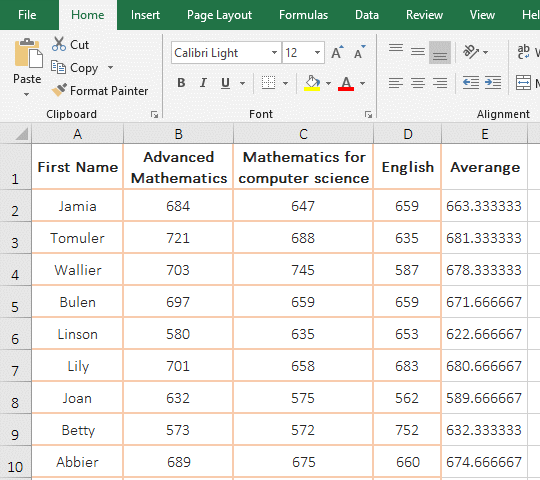Figure 1

(II) Round to 2 decimal places

Select the two cells E3:E4, the current tab is "Home", click the drop-down list box with "General", select "Number" in the pop-up options, the values in E3:E4 are rounded two decimal places, all zeros behind the decimal point are also retained; the operating process steps are shown in Figure 2: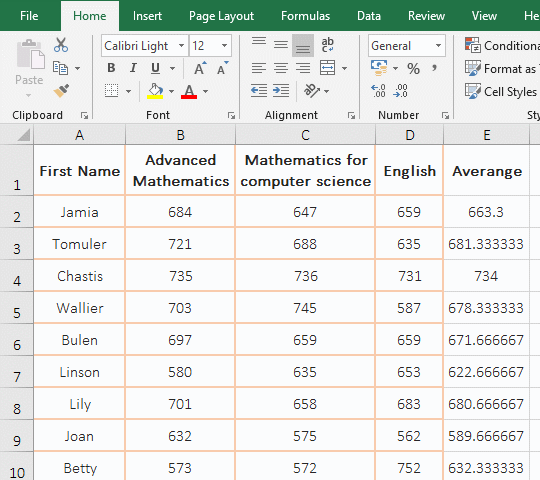Figure 2

(III) Round to 1 decimal place and all zeros behind the decimal point will not be displayed

1. Double-click cell E2, change the formula inside it to =ROUND(AVERAGE(B2:D2),1), press Enter to return the result of averaging with one decimal place 663.3
; Select E2, move the mouse to the cell fill handle in the lower corner of E2, double-click the left button, all the remaining cells in column E will return the result of rounding one decimal place, and all the scores that are 0 behind the decimal point will only retain integers, such as 734 in E4; the operation process steps are shown in Figure 3: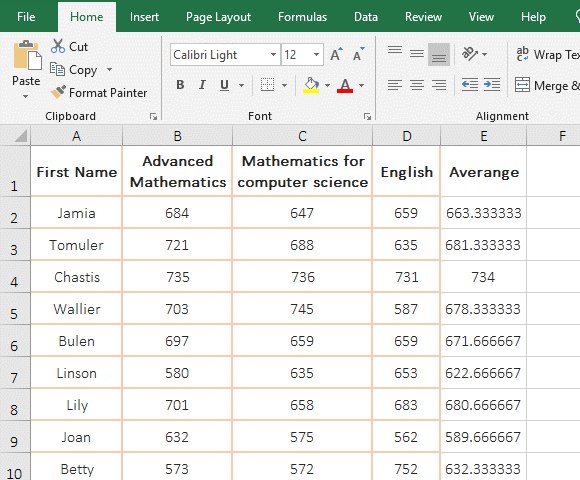Figure 3

2. Formula =ROUND(AVERAGE(B2:D2),1) explanation:

AVERAGE(B2:D2) is used to find the average of all the values in B2:D2, it returns 663.33333333; Round function is used to round to the specified number of decimal places, substitute B2:D2 with the value returned by AVERAGE(B2:D2), the formula becomes =ROUND(663.33333333,1), the second argument 1 means to round to one decimal place, and finally return 663.3.

Hint: If there are errors in retaining decimals, please refer to the article "How to round numbers in excel and fix rounding errors(13 examples, with amount difference 0.1)".

(IV) 0.00 displays 0, when rounds two decimal places in Excel

1. Select all the cells in which you want to round two decimal places(such as A2:A4), press Ctrl + 1 to open the "Format Cells" dialog box, select "Custom", and copy 0.00;-0.00;0 to the textbox under "Type", Click "OK", the selected values are rounded to two decimal places, 0.00 only displays 0; the operation process steps, see screenshot in Figure 4: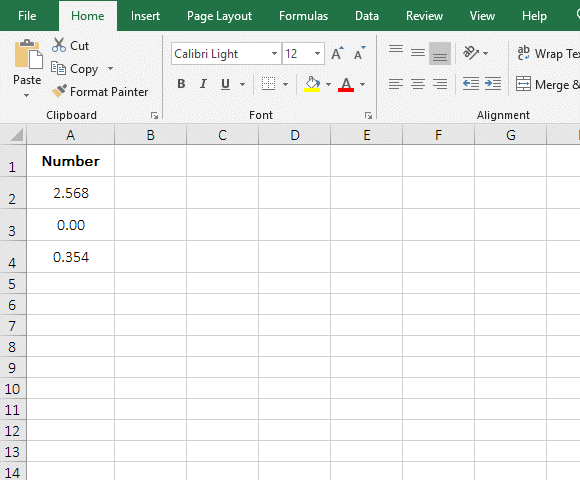Figure 4

2. If you want to round three decimal places, you only need to change 0.00;-0.00;0 to 0.000;-0.000;0, and so on.

### II, 0 is displayed as a dash or not displayed and 0 before the number is displayed in Excel

(I) How to make 0 a dash in excel

1. Select cell D2, hold down "Shift", click D8, select all the cells that you want to display 0 as a dash; press Ctrl + 1, open the "Format Cells" dialog box, select "Number" tab, then select "Custom", copy [=0]"-" to the textbox under "Type", click "OK", 0 will be displayed as a dash(-); the operation process steps, as shown in Figure 5: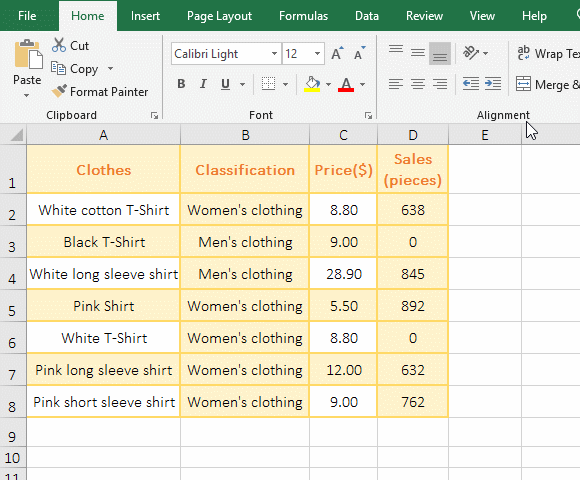Figure 5

2. Tip: If a column of data is set, you can click column number to select a column.

(II) Excel if 0 then blank

1. Select any cell in the table, press Ctrl + F to open the "Find and Replace" dialog box, enter 0 in the textbox to the right of "Find what", and click "Options" to expand; select "By Columns" for "Search", select "Values" for "Look in", check "Match entire cell contents", click "Find All", press Ctrl + A to select all 0, and click "Close" to close the "Find and Replace" dialog box.

2. Press Ctrl + 1, open the "Format Cells" dialog box, select the "Number" tab, then select "Custom" in the lower left corner of the dialog box, and copy [=0]"" to the textbox "Under" "Type" , click "OK", then if 0 then blank, that is, the cell where 0 is located will be left blank; the operation steps are shown in Figure 6: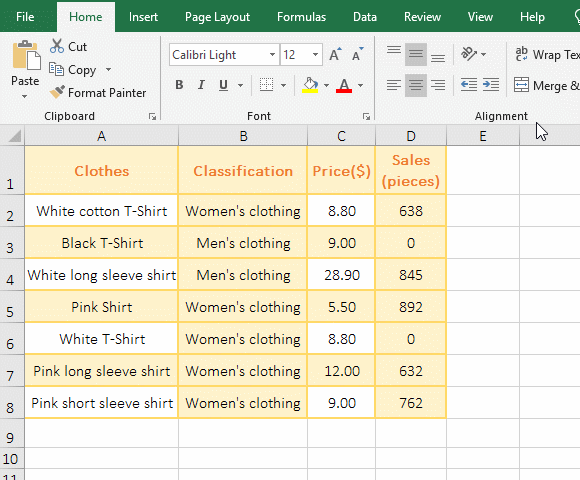Figure 6

If the 0 in the cell is not required to be blank, you only need to set the format to "General" or "Value". The setting method is the same as above.

(III) How to show 0 in excel before numbe(How to stop 0 disappearing in excel)

1. Method 1: Convert numbers into text with single quote.

Select cell A1, enter single quote, then enter the number 0036, and press Enter, the two 0 before the number do not disappear, because the single quote convert the number into text. The operation steps are shown in Figure 7: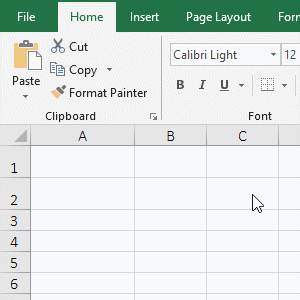Figure 7

2. Method 2: Format the cell as text.

Select A2, press Ctrl + 1, open the "Format Cells" dialog box, select the "Number" tab, then select "Text" on the left, click "OK", enter 00035, and press enter, the three zeros before the number are displayed; the operation process steps are shown in Figure 8: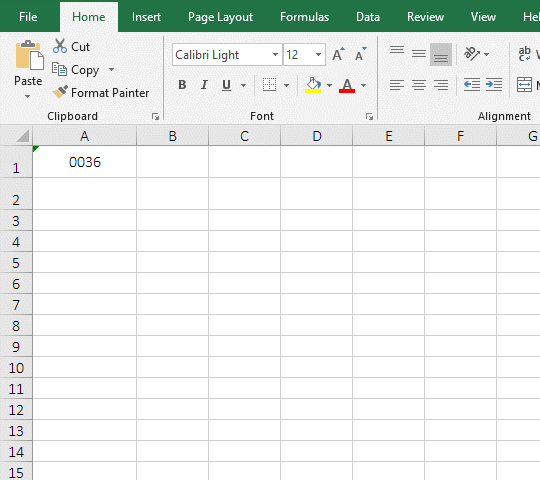Figure 8